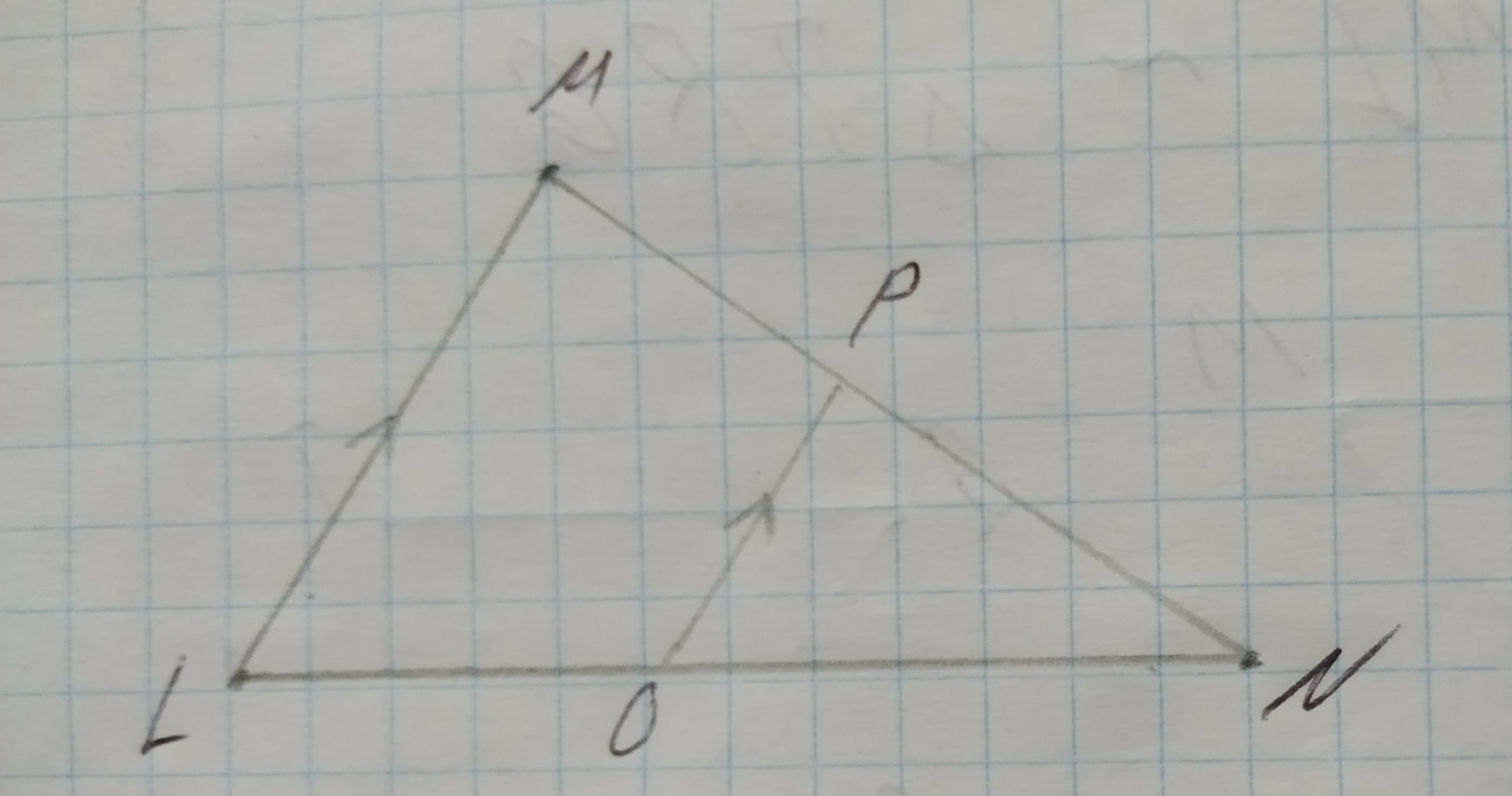# Determine whether the triangles are similar.If so, write a similarity statement and name the postulate or theorem you used. If not, explain.12210107061.jpgKaycee Roche 2021-02-03 Answered

Determine whether the triangles are similar.
If so, write a similarity statement and name the postulate or theorem you used. If not, explain.• Questions are typically answered in as fast as 30 minutes

### Plainmath recommends

• Get a detailed answer even on the hardest topics.
• Ask an expert for a step-by-step guidance to learn to do it yourself.Daphne Broadhurst

Step 1 given
we have been given a triangle MNL in which P is a point on the side MN and O is the point on the side NL and OP is parallel ML
Step 2
first, we will take $$\triangle NPO\ and\ \triangle NML$$
$$\angle N= \angle N$$ (common angle)
$$\angle NPO = \angle NML$$ (corresponding angles as $$OP || ML$$)
so, the AA rule which states in two triangles, corresponding angles are equal, then their corresponding sides are in the same ratio and hence the two triangles are similar.
$$\triangle NML \approx \triangle NPO$$ (AA rule)
therefore the two triangles NML and NPO are similar.